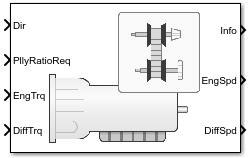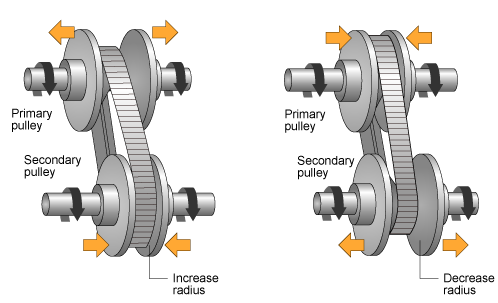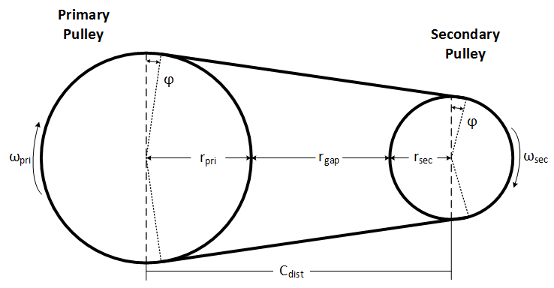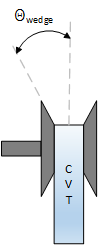# Continuously Variable Transmission

Push belt continuously variable transmission with independent radii control

• Library:
• Powertrain Blockset / Transmission / Transmission Systems

•## Description

The Continuously Variable Transmission block implements a push belt continuously variable transmission (CVT) with independent radii control. Use the block for control system design, powertrain matching, and fuel economy studies. You can configure the block for internal or external control:

• Internal — Input direction and pulley ratio requests

• External — Input direction and pulley displacement requests

The table summarizes the pulley kinematic, speed reduction, and dynamic calculations made by the Continuously Variable Transmission block.

CalculationPulley KinematicsReverse and Final Speed ReductionDynamics
Final angular speed ratio
Belt torque applied to the secondary and primary pulleys
Torque applied to the secondary and primary pulleys
Angular velocity of secondary and primary pulleys
Belt and pulley geometry
Belt linear speed
Wrap angle on secondary and primary pulley

The figure shows the CVT variator with two configurations. In the first configuration, which illustrates speed reduction, the variator is set to decrease the primary pulley radius and increase the secondary pulley radius. In the second configuration, which illustrates overdrive, the variator is set to increase the primary pulley radius and decrease the secondary pulley radius.### Pulley Kinematics

Using the physical dimensions of the system, the block calculates the primary and secondary variator positions that meet the pulley ratio request.

The figure and equations summarize the geometric dependencies.`$\begin{array}{l}{C}_{dist}=r{p}_{max}+{r}_{gap}+{r}_{sec_max}\\ {L}_{0}=f\left(r{p}_{max},r{s}_{max},r{p}_{min},r{s}_{min},{C}_{dist}\right)\\ rati{o}_{command}=f\left(rati{o}_{request},rati{o}_{max},rati{o}_{min}\right)\\ {r}_{pri}=f\left({r}_{0},rati{o}_{command},{C}_{dist}\right)\\ {r}_{\mathrm{sec}}=f\left({r}_{0},rati{o}_{command},{C}_{dist}\right)\\ {x}_{pri}=f\left({r}_{0},{r}_{pri},{\theta }_{wedge}\right)\\ {x}_{\mathrm{sec}}=f\left({r}_{0},{r}_{\mathrm{sec}},{\theta }_{wedge}\right)\end{array}$`

The equations use these variables.

 ratiorequest Pulley gear ratio request ratiocommand Pulley gear ratio command, based on request and physical limitations rgap Gap distance between variator pulleys Cdist Distance between variator pulley centers rpmax Maximum variator primary pulley radius rsmax Maximum variator secondary pulley radius rpmin Minimum variator primary pulley radius rsmin Minimum variator secondary pulley radius ro Initial pulley radii with gear ratio of `1` Lo Initial belt length, resulting from variator specification xpri Variator primary pulley displacement, resulting from controller request xsec Variator secondary pulley displacement, resulting from controller request rpri Variator primary pulley radius, resulting from controller request rsec Variator secondary pulley radius, resulting from controller request Θwedge Variator wedge angle Φ Angle of belt to pulley contact point L Belt length, resulting from variator position

### Reverse and Final Speed Reduction

The CVT input shaft connects to a planetary gear set that drives the primary pulley. The shift direction determines the input gear inertia, efficiency, and gear ratio. The shift direction is the filtered commanded direction:

`$\frac{Di{r}_{shift}}{Dir}\left(s\right)=\frac{1}{{\tau }_{s}s+1}$`

For forward motion ($Di{r}_{shift}=1$):

`$\begin{array}{l}{N}_{i}=1\\ {\eta }_{i}={\eta }_{fwd}\\ {J}_{i}={J}_{fwd}\end{array}$`

For reverse motion ($Di{r}_{shift}=-1$):

`$\begin{array}{l}{N}_{i}=-{N}_{rev}\\ {\eta }_{i}={\eta }_{rev}\\ {J}_{i}={J}_{rev}\end{array}$`

The gear ratio and efficiency determine the input drive shaft speed and torque applied to the primary pulley:

`${T}_{app_pri}={\eta }_{i}{N}_{i}{T}_{i}$`

The block reduces the secondary pulley speed and applied torque using a fixed gear ratio.

`$\begin{array}{l}{T}_{app_sec}=\frac{{T}_{o}}{{\eta }_{o}{N}_{o}}\\ {\omega }_{o}=\frac{{\omega }_{sec}}{{N}_{o}}\end{array}$`

The final gear ratio, without slip, is given by:

`${N}_{final}=\frac{{\omega }_{i}}{{\omega }_{o}}={N}_{i}{N}_{o}\frac{{r}_{sec}}{{r}_{pri}}$`

The equations use these variables.

 Ni Input planetary gear ratio Dir CVT direction command Dirshift Direction used to determine planetary inertia, efficiency, and ratio τs Direction shift time constant ηfwd, ηrev Forward and reverse gear efficiency, respectively Jfwd, Jrev Forward and reverse gear inertia, respectively Nrev Reverse gear ratio Tapp_pri, Tapp_sec Torque applied to primary and secondary pulleys, respectively Ti Input drive shaft torque ωi, ωo Input and output drive shaft speed, respectively ωpri, ωsec Primary and secondary pulley speed, respectively Nfinal Total no-slip gear ratio

### Dynamics

The maximum torque that the CVT can transmit depends on the friction between the pulleys and belt. According to Prediction of Friction Drive Limit of Metal V-Belt, the torque friction is defined as:

`${T}_{fric}\left({r}_{p},\mu \right)=\frac{2\mu {F}_{ax}{r}_{p}}{\mathrm{cos}\left({\vartheta }_{wedge}\right)}$`

Without macro slip, the tangential acceleration of the pulley is assumed to be equal to the belt acceleration. Once the torque reaches the static friction limit, the belt begins to slip, and the pulley and belt acceleration are independent. During slip, the torque transmitted by the belt is a function of the kinetic friction factor. During the transition from slip to non-slip conditions, the belt and tangential pulley velocities are equal.

The block implements these equations for four different slip conditions.

ConditionEquations

Belt slips on both secondary and primary pulleys

`$\begin{array}{l}\left({J}_{pri}+{J}_{i}\right){\stackrel{˙}{\omega }}_{pri}={T}_{app_pri}-{T}_{BoP_pri}-{b}_{pri}{\omega }_{pri}\\ {J}_{sec}{\stackrel{˙}{\omega }}_{sec}={T}_{app_sec}-{T}_{BoP_sec}-{b}_{sec}{\omega }_{sec}\\ {m}_{b}{\stackrel{˙}{v}}_{b}=\frac{{T}_{BoP_pri}}{{r}_{pri}}+\frac{{T}_{BoP_sec}}{{r}_{sec}}-{b}_{b}{v}_{b}\\ {r}_{pri}{\omega }_{pri}\ne {v}_{b}\\ {r}_{sec}{\omega }_{sec}\ne {v}_{b}\end{array}$`

Belt slips on only the primary pulley

`$\begin{array}{l}\left({J}_{pri}+{J}_{i}\right){\stackrel{˙}{\omega }}_{pri}={T}_{app_pri}-{T}_{BoP_pri}-{b}_{pri}{\omega }_{pri}\\ \left({m}_{b}+\frac{{J}_{sec}}{{r}^{2}{}_{sec}}\right){\stackrel{˙}{v}}_{b}=\frac{{T}_{BoP_pri}}{{r}_{pri}}+\frac{{T}_{BoP_sec}}{{r}_{sec}}-\left({b}_{b}+\frac{{b}_{sec}}{{r}^{2}{}_{sec}}\right){v}_{b}\\ {\omega }_{sec}=\frac{{v}_{b}}{{r}_{sec}}\\ {r}_{pri}{\omega }_{pri}\ne {v}_{b}\\ {T}_{BoP_pri}=\mathrm{sgn}\left({r}_{pri}{\omega }_{pri}-{v}_{b}\right){T}_{fric}\left({r}_{pri},{\mu }_{kin}\right)\\ |{T}_{BoP_sec}|<{T}_{fric}\left({r}_{sec},{\mu }_{static}\right)\end{array}$`

Belt slips on only the secondary pulley

`$\begin{array}{l}\left({m}_{b}+\frac{{J}_{pri}+{J}_{i}}{{r}^{2}{}_{pri}}\right){\stackrel{˙}{v}}_{b}=\frac{{T}_{app_pri}}{{r}_{pri}}+\frac{{T}_{BoP_sec}}{{r}_{sec}}-\left({b}_{b}+\frac{{b}_{pri}}{{r}^{2}{}_{pri}}\right){v}_{b}\\ {J}_{sec}{\stackrel{˙}{\omega }}_{b}={T}_{app_sec}+{T}_{BoP_sec}-{b}_{sec}{\omega }_{sec}\\ {\omega }_{pri}=\frac{{v}_{b}}{{r}_{pri}}\\ {r}_{sec}{\omega }_{sec}\ne {v}_{b}\\ {T}_{BoP_sec}=\mathrm{sgn}\left({r}_{sec}{\omega }_{sec}-{v}_{b}\right){T}_{fric}\left({r}_{sec},{\mu }_{kin}\right)\\ |{T}_{BoP_pri}|<{T}_{fric}\left({r}_{pri},{\mu }_{static}\right)\end{array}$`

Belt does not slip

`$\begin{array}{l}\left({m}_{b}+\frac{{J}_{sec}}{{r}^{2}{}_{sec}}+\frac{{J}_{pri}+{J}_{i}}{{r}^{2}{}_{pri}}\right){\stackrel{˙}{v}}_{b}=\frac{{T}_{app_pri}}{{r}_{pri}}+\frac{{T}_{app_sec}}{{r}_{sec}}-\left({b}_{b}+\frac{{b}_{sec}}{{r}^{2}{}_{sec}}+\frac{{b}_{pri}}{{r}^{2}{}_{pri}}\right){v}_{b}\\ {\omega }_{pri}=\frac{{v}_{b}}{{r}_{pri}}\\ {\omega }_{sec}=\frac{{v}_{b}}{{r}_{sec}}\\ |{T}_{BoP_pri}|<{T}_{fric}\left({r}_{pri},{\mu }_{static}\right)\\ |{T}_{BoP_sec}|<{T}_{fric}\left({r}_{sec},{\mu }_{static}\right)\end{array}$`

Slip direction

`$\begin{array}{l}PriSlipDir=\left\{\begin{array}{cc}0& {r}_{pri}{\omega }_{pri}={v}_{b}\\ 1& {r}_{pri}{\omega }_{pri}>{v}_{b}\\ -1& {r}_{pri}{\omega }_{pri}<{v}_{b}\end{array}\\ SecSlipDir=\left\{\begin{array}{cc}0& {r}_{sec}{\omega }_{sec}={v}_{b}\\ 1& {r}_{sec}{\omega }_{sec}>{v}_{b}\\ -1& {r}_{sec}{\omega }_{sec}<{v}_{b}\end{array}\end{array}$`

The equations use these variables.

 TBoP_pri, TBoP_sec Belt torque acting on the primary and secondary pulleys, respectively Tapp_pri, Tapp_sec Torque applied to primary and secondary pulleys, respectively Jpri, Jsec Primary and secondary pulley rotational inertias, respectively bpri, bsec Primary and secondary pulley rotational viscous damping, respectively Fax Pulley clamp force μ Coefficient of friction μkin, μstatic Coefficient of kinetic and static friction vb, аb Linear speed and acceleration of the belt, respectively mb Total belt mass rpri, rsec Radii of the primary and secondary pulleys, respectively Φwrap Wrap angle of belt to pulley contact point Φwrap_pri, Φwrap_sec Primary and secondary pulley wrap angles, respectively

### Power Accounting

For the power accounting, the block implements these equations.

Bus Signal DescriptionVariableEquations

`PwrInfo`

`PwrTrnsfrd` — Power transferred between blocks

• Positive signals indicate flow into block

• Negative signals indicate flow out of block

`PwrEng`

Engine power

Peng

${\omega }_{i}{T}_{i}$
`PwrDiffrntl`

Differential power

Pdiff

${\omega }_{o}{T}_{o}$

`PwrNotTrnsfrd` — Power crossing the block boundary, but not transferred

• Positive signals indicate an input

• Negative signals indicate a loss

`PwrBltLoss`

Belt slip power loss

Pbltloss

$\begin{array}{l}\left({J}_{in}+{J}_{pri}\right){\stackrel{˙}{\omega }}_{pri}{\omega }_{pri}+\\ {J}_{sec}{\stackrel{˙}{\omega }}_{sec}{\omega }_{sec}+\\ {m}_{b}{\stackrel{˙}{v}}_{b}{v}_{b}+{b}_{pri}{\omega }_{pri}^{2}+{b}_{sec}{\omega }_{sec}^{2}+{b}_{b}{v}_{b}^{2}-\\ {T}_{ap{p}_{pri}}{\omega }_{pri}-{T}_{ap{p}_{sec}}{\omega }_{sec}\end{array}$
`PwrGearInLoss`

Input planetary gear mechanical power loss

Pgrinloss

$-|{\omega }_{i}{T}_{i}-{Τ}_{app_pri}{\omega }_{pri}|$
`PwrGearOutLoss`

Output gear reduction mechanical power loss

Pgroutloss

$-|{\omega }_{o}{T}_{o}-{Τ}_{app_sec}{\omega }_{sec}|$

`PwrDampLoss`

Mechanical damping loss

Pdamploss

$-{b}_{pri}{\omega }_{pri}^{2}-{b}_{sec}{\omega }_{sec}^{2}-{b}_{b}{v}_{b}^{2}$

`PwrStored` — Stored energy rate of change

• Positive signals indicate an increase

• Negative signals indicate a decrease

`PwrStoredTrans`

Rate change in rotational kinetic energy

Pstr

$\left({J}_{in}+{J}_{pri}\right){\stackrel{˙}{\omega }}_{pri}{\omega }_{pri}+{J}_{sec}{\stackrel{˙}{\omega }}_{sec}{\omega }_{sec}+{m}_{b}{\stackrel{˙}{v}}_{b}{v}_{b}$

The equations use these variables.

 Tapp_pri, Tapp_sec Torque applied to primary and secondary pulleys, respectively Ti, To Input and output drive shaft torque, respectively Jpri, Jsec Primary and secondary pulley rotational inertias, respectively bpri, bsec Primary and secondary pulley rotational viscous damping, respectively ωpri, ωsec Primary and secondary pulley speed, respectively ωi, ωo Input and output drive shaft speed, respectively vb, аb Linear speed and acceleration of the belt, respectively rpri, rsec Radii of the primary and secondary pulleys, respectively

## Ports

### Inputs

expand all

Direction request, Dirreq, controlling the direction. The block filters the request to determine the direction, forward or reverse. Dir equals `1` for forward motion. Dir equals `-1` for reverse.

CVT pulley ratio request, ratiorequest.

#### Dependencies

To create this port, for the Control mode parameter, select ```Ideal integrated controller```.

Variator primary pulley displacement, xpri, in m.

#### Dependencies

To create this port, for the Control mode parameter, select ```External control```.

Variator secondary pulley displacement, xsec, in m.

#### Dependencies

To create this port, for the Control mode parameter, select ```External control```.

External torque applied to the input drive shaft, Ti, in N·m.

External torque applied to the output drive shaft, To, in N·m.

### Output

expand all

Bus signal containing these block calculations.

SignalDescriptionVariableUnits

`EngTrq`

Input shaft torque

Ti

N·m

`DiffTrq`

Output shaft torque

To

N·m

`EngSpd`

Input shaft speed

ωi

`DiffSpd`

Output shaft speed

ωo

`PriRadius`

rpri

m

`PriPhi`

Primary pulley wrap angle

Φpri

`SecRadius`

rsec

m

`SecPhi`

Secondary pulley wrap angle

Φsec

`BltLngthDelta`

Change in belt length

ΔL

m

`BltLngth`

Belt length

L

m

`BltLngthInit`

Initial belt length

Lo

m

`BltOnPriTrq`

Belt torque acting on the primary pulley

TBoP_pri

N·m

`BltOnSecTrq`

Belt torque acting on the secondary pulley

TBoP_sec

N·m

`BltVel`

Linear speed of the belt

vb

m/s

`PriAngVel`

Primary pulley speed

ωpri

`SecAngVel`

Secondary pulley speed

ωsec

`PriSlipDir`

Primary pulley slip direction indicator

PriSlipDir

N/A

`SecSlipDir`

Secondary pulley slip direction indicator

SecSlipDir

N/A

`TransSpdRatio`

Total no-slip gear ratio

Nfinal

N/A

`PwrInfo`

`PwrTrnsfrd`

`PwrEng`

Engine power

Peng

W
`PwrDiffrntl`

Differential power

Pdiff

W
`PwrNotTrnsfrd``PwrBltLoss`

Belt slip power loss

Pbltloss

W
`PwrGearInLoss`

Input planetary gear mechanical power loss

Pgrinloss

W
`PwrGearOutLoss`

Output gear reduction mechanical power loss

Pgroutloss

W
`PwrDampLoss`

Mechanical damping loss

Pdamploss

W
`PwrStored``PwrStoredTrans`

Rate change in rotational kinetic energy

Pstr

W

Input drive shaft angular speed, ωi, in rad/sec.

Output drive shaft angular speed, ωo, in rad/sec.

## Parameters

expand all

Specify the control method, either internal or external.

#### Dependencies

This table summarizes the port and input model configurations.

Control ModeCreates Ports
```Ideal integrated controller```

`PllyRatioReq`

```External control```

`PriDisp`

`SecDisp`

Kinematics

Maximum variator primary pulley radius, rpmax, in m.

Maximum variator secondary pulley radius, rsmax, in m.

Minimum variator primary pulley radius, rpmin, in m.

Minimum variator secondary pulley radius, rsmin, in m.

The gap between the secondary and primary pulleys, rgap, in m. The figure shows the pulley geometry.Variator wedge angle, Θwedge, in deg.Dynamics

Primary pulley inertia, Jpri, in kg·m^2.

Secondary pulley inertia, Jsec, in kg·m^2.

Primary pulley damping coefficient, bpri, in N·m·s/rad.

Secondary pulley damping coefficient, bsec, in N·m·s/rad.

Belt damping coefficient, bb, in kg/s.

Static friction coefficient between the belt and primary pulley, μstatic, dimensionless.

Kinetic friction coefficient between the belt and primary pulley, μkin, dimensionless.

Belt mass, mb, in kg.

Pulley clamp force, Fax, in N.

Reverse and Output Ratio

Forward inertia, Jfwd, in kg·m^2.

Reverse inertia, Jrev, in kg·m^2.

Forward efficiency, ηfwd, dimensionless.

Reverse efficiency, ηrev, dimensionless.

Reverse gear ratio, Nrev, dimensionless.

Shift time constant, τs, in s.

Output gear ratio, No, dimensionless.

Output gear efficiency, ηo, dimensionless.

 Ambekar, Ashok G. Mechanism and Machine Theory. New Delhi: Prentice-Hall of India, 2007.

 Bonsen, B. Efficiency optimization of the push-belt CVT by variator slip control. Ph.D. Thesis. Eindhoven University of Technology, 2006.

 CVT How Does It Work. CVT New Zealand 2010 Ltd, 10 Feb. 2011. Web. 25 Apr. 2016.

 Klaassen, T. W. G. L. The Empact CVT: Dynamics and Control of an Electromechanically Actuated CVT. Ph.D. Thesis. Eindhoven University of Technology, 2007.

 Sakagami, K. Prediction of Friction Drive Limit of Metal V-Belt. Warrendale, PA: SAE International Journal of Engines 8(3):1408-1416, 2015.

## SupportGet trial now